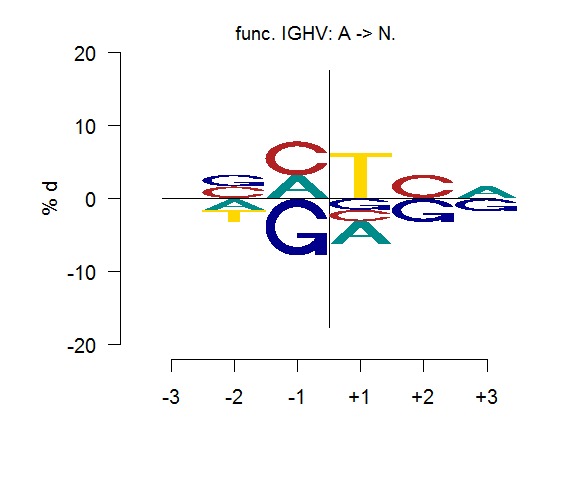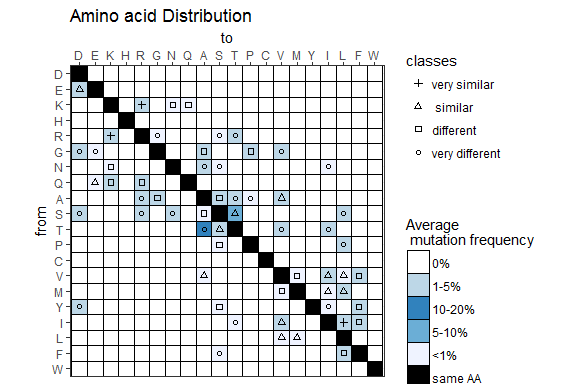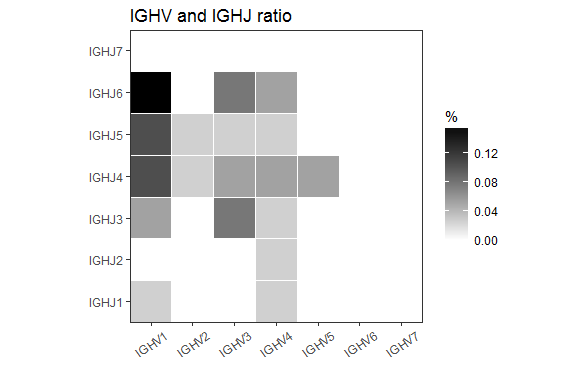BCellMA: B Cell Receptor Somatic Hyper Mutation Analysis

Introduction

The BCellMA package includes a set of functions to analyze for instance nucleotide frequencies as well as transition and transversion. BCellMA can reconstruct germline sequences based on IMGT outputs, calculate and plot the difference (%) of nucleotides at 6 positions around a mutation to identify and characterize hotspot motifs as well as calculate and plot average mutation frequencies of nucleotide mutations resulting in amino acid substitution.

# Load required packages
library("BCellMA")

Example data

An Ig gene database example using IMGT/HighV-QUEST is included in the BCellMA package.

The Ig sequences were taken from the following publication:

Mueller A. Christoph Brieske C. Schinke S. Csernok E. Gross WL. Hasselbacher K. Voswinkel J. and Holl-Ulrich K. Plasma cells within granulomatous inflammation display signs pointing to autoreactivity and destruction in granulomatosis with polyangiitis. Arthritis Res Ther 16(1):R55, 2014.

IMGT/HighV-QUEST reference:

Brochet X. Lefranc MP. and Giudicelli V. IMGT/V-QUEST: the highly customized andintegrated system for IG and TR standardized V-Jand V-D-J sequence analysis.NucleicAcids Res., 36(Web Server issue):W503–W508, 2008. doi: 10.1093/nar/gkn316.

Data from the Excel file 2 are named “IMGT-gapped-nt-sequences” and are included in IMGTtab2. Data from the Excel file 7 are called “The V-REGION-mutation-table” and are included in IMGTtab7. Data from the Excel file 8 are called “The V-REGION-nt-mutation-statistics” and are included in IMGTtab8.

# Subset example data
data("IMGTtab1")
data("IMGTtab2")
data("IMGTtab7")
data("IMGTtab8")

Functions to analyze nucleotide frequencies as well as transition and transversion mutations

The function nucleotide_mutation() can be used to calculate the number of nucleotide mutations, transitions and transversions. Nucleotide mutation can be plotted as a pie plot. Transition and transversion mutations can be plotted as a bar plot. For more information see ?nucleotide_mutation.

bm_proband<- nucleotide_mutation(IMGTtab8[,11:22])

Calculation and plotting of hotspot motifs

For the calculation of hotspot motifs the germline genes are required. GermlineReconstr() is a function that reconstructs germline gene sequences based on IMGT outputs.

germline<-germlineReconstr(IMGTtab2$V_REGION, IMGTtab7$V_REGION)

The function na_funktion() checks for reconstruction errors in the Ig sequences. It controls for the presence of missing values (“NA”) in a sequence by counting NAs. If the answer is null, then there are no errors in the germline reconstruction. If the answer is different from null, the mutated Ig sequence must be checked manually.

na_funktion(germline)
##  0

Calculation of the total nucleotide number three positions before and after a mutation with the function hotspotmutMatrix().

data<-targetingMatrix(data_tab2=IMGTtab2, data_tab_germline=germline, data_tab7=IMGTtab7)

data$mutzur_A ## Pos-3 Pos-2 Pos-1 Pos+1 Pos+2 Pos+3 ## A 10 14 16 4 9 16 ## C 14 21 20 12 24 13 ## G 18 18 11 19 19 14 ## T 17 6 14 26 7 12 A target motif describes a short DNA motif in which mutations are preferentially inserted. The change around the mutated nucleotide flanking three bases each is expressed as the difference (%) between the substitution frequency in the mutated sequence and the substitution frequency in the germline sequence with the function targeting_motiv(). The substitution frequency in the mutated sequence is calculated as: $SFM_{B}^{i}= \frac{n_{B}^{i}}{ n_{A}^{i} + n_{T}^{i} + n_{C}^{i} +n_{G}^{x_i}}.$ The substitution frequency in the germline sequence is calculated as: $SFG_{B}^{i}= \frac{n_{B}^{i}}{ n_{A}^{i} + n_{T}^{i} + n_{C}^{i} +n_{G}^{i}},$ $$n_{B}^{i}$$ is the number of nucleotides $$B=\{A, T, C, G\}$$ at the position $$i= \{-3, -2, -1, 1, 2, 3\}$$. Reference: Spencer J. and Dunn-Walters DK. Hypermutation at A-T base pairs: the A nucleotidereplacement spectrum is affected by adjacent nucleotides and there is no reverse comple-mentary of sequences flanking muated A and T nucleotides.J Immunol, 175(8):5170 - 5177,2005. Zuckerman NS., Hazanov H., Barak M., Edelman H., Hess S., Shcolnik H., Dunn-Walters D.,and Mehr R. Somatic hypermutation and antigen-driven selection of B cells are altered inautoimmune diseases.J Autoimmun, 35(4):325 - 335, 2010. doi: 10.1016/j.jaut.2010.07.004. targeting_motiv_data<-targeting_motiv(data) targeting_motiv_data$A
##   -3        -2        -1        +1        +2        +3
## A  0 -1.694915  3.278689 -3.278689  0.000000  1.818182
## C  0  1.694915  4.918033 -1.639344  3.389831  0.000000
## G  0  1.694915 -8.196721 -1.639344 -3.389831 -1.818182
## T  0 -1.694915  0.000000  6.557377  0.000000  0.000000

The target motifs can be plotted using the function targeting_motive_plot().

targeting_motive_plot(targeting_motiv_data$A, xfontsize = 15, yfontsize = 15, xlim=20 ) grid.text("func. IGHV: A -> N.", x=0.55, y=unit(20,"lines"), gp=gpar(fontsize=14))Calculating and plotting of average mutation frequencies of nucleotide mutations resulting in amino acid substitution The average amino acid mutation frequency in all regions can be calculated with the function aa_dist(). The average mutation frequency leading to an amino acid exchange is calculated as: $F_{l}(AA_i > AA_j) = \frac{1}{n} \sum_{k=0}^n \frac{M_{l,k}( AA_i > AA_j )}{ \sum_{l=0}^{380} M_{l,k} (AA_i > AA_j )}, i\neq j, 1= 1, 2, ..., 20, j= 1, 2, ..., 19.$. In a group all Ig sequences $$k$$ = 1, 2, …, n are considered. $$M_{l,k}$$ is determined as the number of the specific amino acid exchanges $$l$$ = 1, 2, …, 380 in the Ig sequence $$k$$ from one of the 20 amino acids $$i$$ to another amino acid $$j$$. This specific amino acid exchange number is divided by the sum of all amino acid exchanges $$M_{l,k} (AA_i > AA_j )$$ in the $$k$$-th Ig sequence to obtain relative amino acid exchange value. The average amino acid mutation frequency results from an average value of the relative amino acid exchange values over all $$n$$ Ig sequences. Regions<-cbind(IMGTtab7$FR1_IMGT,
IMGTtab7$CDR1_IMGT, IMGTtab7$FR2_IMGT,
IMGTtab7$CDR2_IMGT, IMGTtab7$FR3_IMGT,
IMGTtab7$CDR3_IMGT) Regions_matrix<-aa_dist(data=Regions) Regions_matrix[1:3,1:17] ## D E K H R G N Q A S T P C V M Y I ## W 0 0 0 0 0 0 0 0 0 0.000000000 0 0 0 0.000000000 0.000000000 0 0 ## F 0 0 0 0 0 0 0 0 0 0.004115226 0 0 0 0.000000000 0.000000000 0 0 ## L 0 0 0 0 0 0 0 0 0 0.000000000 0 0 0 0.009876543 0.006944444 0 0 The average mutation frequency leading to an amino acid exchange can be plotted as an aa_plot(). IMGT/HighV-QUEST classifies amino acid exchanges into four classes according to the change in chemical properties, hydropathy and volume. In the BCellMA package these classes are given by “data Klassen”. data(Klassen) p1<-aa_plot(Regions_matrix, "Amino acid Distribution", "right", Klassen)The average mutation frequency leading to an amino acid exchange can be computed for CDRs only and/or for FRs only. An example is shown below. CDRs<-cbind(IMGTtab7$CDR1_IMGT,
IMGTtab7$CDR2_IMGT, IMGTtab7$CDR3_IMGT)
CDRs_matrix<-aa_dist(data=Regions)
p2<-aa_plot(CDRs_matrix, "Amino acid Distribution", "right", Klassen)Calculating and plotting of average mutation frequencies of nucleotide mutations resulting in amino acid substitution in the CDR3

The average mutation frequency leading to amino acid exchanges in the CDR3 can be calculated with the function aa_cdr3_dist().

family2 = IMGTtab1$J_GENE_and_allele) gane_comb ## IGHV1 IGHV2 IGHV3 IGHV4 IGHV5 IGHV6 IGHV7 ## IGHJ1 0.02631579 0.00000000 0.00000000 0.02631579 0.00000000 0 0 ## IGHJ2 0.00000000 0.00000000 0.00000000 0.02631579 0.00000000 0 0 ## IGHJ3 0.05263158 0.00000000 0.07894737 0.02631579 0.00000000 0 0 ## IGHJ4 0.10526316 0.02631579 0.05263158 0.05263158 0.05263158 0 0 ## IGHJ5 0.10526316 0.02631579 0.02631579 0.02631579 0.00000000 0 0 ## IGHJ6 0.15789474 0.00000000 0.07894737 0.05263158 0.00000000 0 0 ## IGHJ7 0.00000000 0.00000000 0.00000000 0.00000000 0.00000000 0 0 Distribution patterns and frequencies of two genes can be plotted as an gene_comb_plot() gene_comb_plot(gane_comb, "IGHV and IGHJ ratio", legend_position = "right", a = 35, b = 0.5)CDR3 length of IGHV genes Frequency distribution of the CDR3 length can be estimated with lengthCDR3() lenght_tab<-lengthCDR3(as.numeric(IMGTtab1$CDR3_IMGT_length))
lenght_tab
##         7  8  9       10  11       12       13       14       15       16
##  2.631579  0  0 7.894737   0 5.263158 7.894737 15.78947 15.78947 2.631579
##        17       18       19       20       21  22  23       24  25
##  2.631579 10.52632 7.894737 7.894737 7.894737   0   0 2.631579   0
##        26
##  2.631579